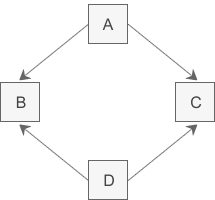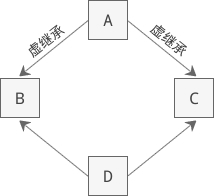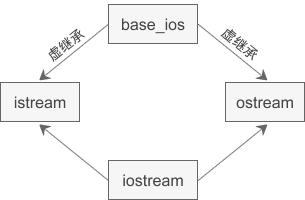# C++虚基类与虚继承

## 为什么有虚继承1. //间接基类A
2. class A{
3. protected:
4. int m_a;
5. };
6. //直接基类B
7. class B: public A{
8. protected:
9. int m_b;
10. };
11. //直接基类C
12. class C: public A{
13. protected:
14. int m_c;
15. };
16. //派生类D
17. class D: public B, public C{
18. public:
19. void seta(int a){ m_a = a; } //命名冲突
20. void setb(int b){ m_b = b; } //正确
21. void setc(int c){ m_c = c; } //正确
22. void setd(int d){ m_d = d; } //正确
23. private:
24. int m_d;
25. };
26. int main(){
27. D d;
28. return 0;
29. }

1. void seta(int a){ B::m_a = a; }

1. void seta(int a){ C::m_a = a; }

1. //间接基类A
2. class A{
3. protected:
4. int m_a;
5. };
6. //直接基类B
7. class B: virtual public A{ //虚继承
8. protected:
9. int m_b;
10. };
11. //直接基类C
12. class C: virtual public A{ //虚继承
13. protected:
14. int m_c;
15. };
16. //派生类D
17. class D: public B, public C{
18. public:
19. void seta(int a){ m_a = a; } //正确
20. void setb(int b){ m_b = b; } //正确
21. void setc(int c){ m_c = c; } //正确
22. void setd(int d){ m_d = d; } //正确
23. private:
24. int m_d;
25. };
26. int main(){
27. D d;
28. return 0;
29. }C++标准库中的 iostream 类就是一个虚继承的实际应用案例。iostream 从 istream 和 ostream 直接继承而来，而 istream 和 ostream 又都继承自一个共同的名为 base_ios 的类，是典型的菱形继承。此时 istream 和 ostream 必须采用虚继承，否则将导致 iostream 类中保留两份 base_ios 类的成员。• 如果 B 和 C 中都没有 x 的定义，那么 x 将被解析为 B 的成员，此时不存在二义性。
• 如果 B 或 C 其中的一个类定义了 x，也不会有二义性，派生类的 x 比虚基类的 x 优先级更高。
• 如果 B 和 C 中都定义了 x，那么直接访问 x 将产生二义性问题。

01-21609
06-031万+03-31895
04-187360
08-31859
07-23100
03-222216
06-1673
09-11
09-29